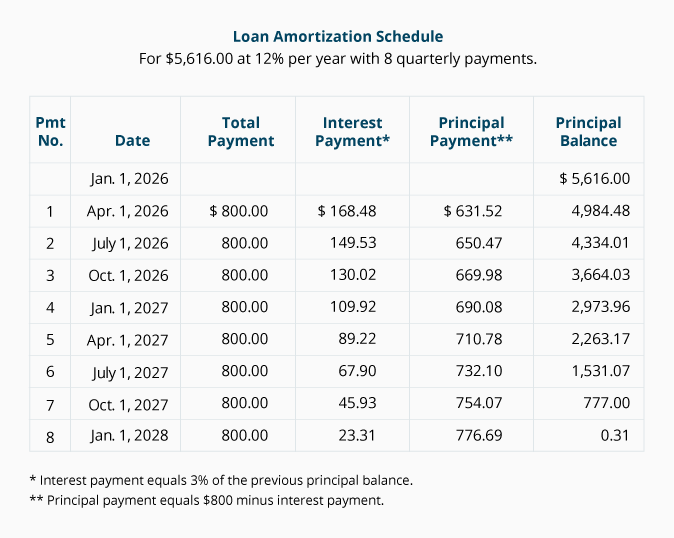# Calculating the Rate (i) in an Ordinary Annuity

Using the PVOA equation, we can calculate the interest rate (i) needed to discount a series of equal payments back to the present value. In order to solve for (i), we need to know the present value amount, the amount of the equal payments, and the length of time (n).

### Exercise #9

Sylvia has an investment account that shows a balance of \$2,523.50 on January 1, 2023. She wants to make five withdrawals of \$700 each on December 31 of years 2023 through 2027. Sylvia wants the account to have a balance of \$0 on December 31, 2027. In order to proceed with her plans, what annual interest rate does Sylvia need on her account, assuming that annual interest earnings are added to the principal on December 31 of each year?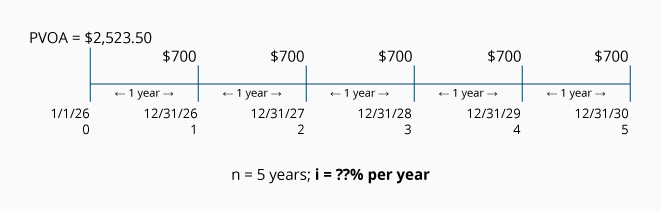Calculation of Exercise #9 using the PVOA Table

The interest rate for the ordinary annuity described above can be computed with the following equation: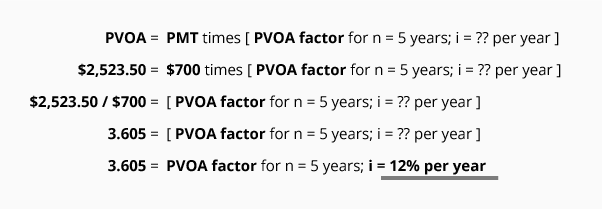Let's review this calculation. We insert into the equation the components that we know: the present value, payment amount, and the number of periods. In line four, we calculate our factor to be 3.605. We now know both the PVOA factor (3.605) and the number of years (n = 5). We go to the PVOA Table and look across the n = 5 row until we come to the factor 3.605. Tracking up the column, we see that the factor is in the column with the heading of 12%. Since the periods in question are annual periods, the answer of i = 12% means the investment has to earn 12% per year.

Here is the proof of this answer:

Investment Account Activity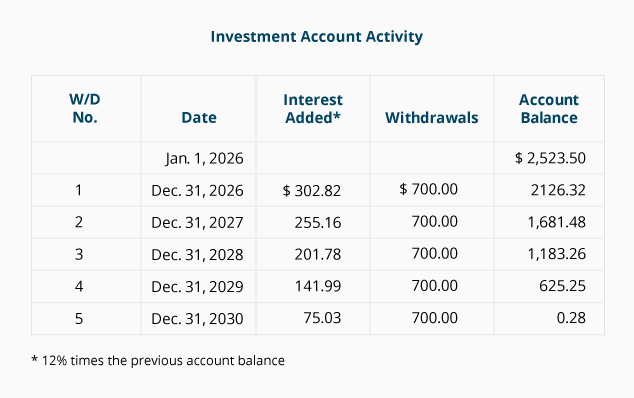### Exercise #10

Matt is moving to Texas and needs to borrow \$5,616 on January 1, 2023. His budget will allow him to make quarterly payments of \$800 on the first day of January, April, July, and October. Matt's loan includes 8 quarterly payments; the first payment is due on April 1, 2023. What is the rate (compounded quarterly) that Matt will be paying (and the lender will be receiving) under this arrangement?

Before calculating the interest rate, we organize the information on a timeline: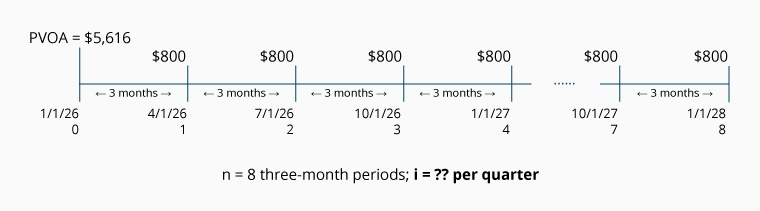Calculation of Exercise #10 using the PVOA Table

The number of periods/payments in the ordinary annuity described above can be computed with the following PVOA equation: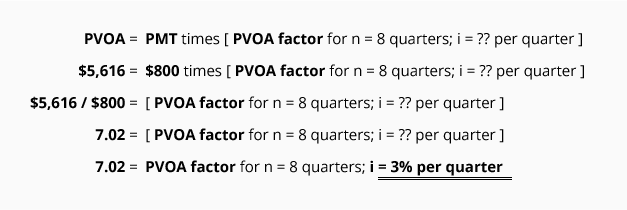Let's review this calculation. We insert into the equation the components that we know: the present value, the recurring payment amount, and the number of periods. In line four, we calculate our factor to be 7.02. We now know both the PVOA factor (7.02) and the number of periods (n = 8). We go to the PVOA Table and look across the n = 8 row until we come to the factor 7.02. Tracking up the column, we see that we are in the 3% column. Since the periods in question are quarterly periods, the answer of i = 3% means the loan has an annual rate of 12% per year (3% times 4 quarters per year).

Here is the proof of this calculation:

Loan Amortization Schedule
(For \$5,616.00 at 12% per year with 8 quarterly payments.)